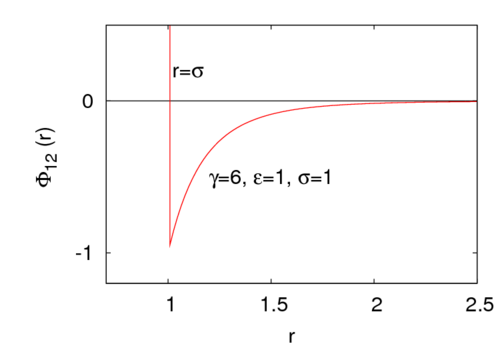# Sutherland potential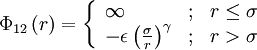$\Phi_{12}\left( r \right) = \left\{ \begin{array}{lll} \infty & ; & r \leq \sigma \\ - \epsilon\left( \frac{ \sigma }{r}\right)^{\gamma} & ; & r > \sigma \end{array} \right.$
where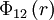$\Phi_{12}\left( r \right)$ is the intermolecular pair potential,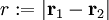$r := |\mathbf{r}_1 - \mathbf{r}_2|$ is the distance between site 1 and site 2,$\sigma$ is the radius of the central hard core,$\epsilon$ is the energy well depth (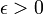$\epsilon > 0$), and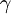$\gamma$ is a parameter that controls the interaction range. For example, for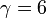$\gamma=6$ the potential look like